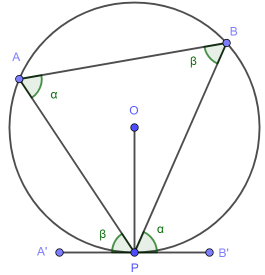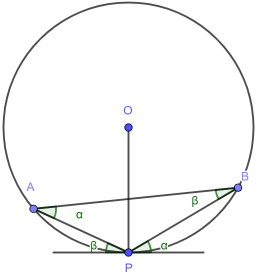# Circle theorems: alternate segment

1. Thales. The easiest circle theorem.
2. Inscribed angle. The angle subtending a major arc is constant.
3. Cyclic quadrilateral - opposite angles sum to 180°. Plus minor arc.
4. Tangent radius. Blindingly obvious, but a slippery proof.
5. Alternate segment. Perhaps the most unintuitive.
6. Chord bisection by radius. The relevant angle is 90°.
7. Equal length tangents. For completeness.

## Statement and diagram

The alternate segment theorem states that the angle between the tangent and chord equals the angle in the alternate segment. The alternate segment? A diagram can help unpack that.

Let’s draw:

• a circle, centre O
• a point P on its circumference
• a tangent to the circle at P

Now connect:

• O and P to form a radius
• P, A and B to form a triangleThe chord PA divides the circle into a major segment and a minor segment. There is an angle in each and the alternate segment theorem says these are equal - in our diagram to β.

Similarly, the chord PB divides the circle into major minor segments and the highlighted angles are equal - to α, say.

The “most opposite” angles are equal.

## Important notes to the diagram

The diagram is usually drawn as above but in general:

• the circle centre O does not have to be inside ΔPAB
• the point P does not have to be on the major segment

We can therefore pull A and B down, so that neither of the above hold and the angle equivalence will remain, although the values of α and β will of course change.## Try it yourself

This is perhaps the least intuitive of the circle theorems. Have a play!

## Proof

We join the points O and B with a line.From the inscribed angle theorem we know that as we move A the ∠PAB remains constant and in fact equals ∠POB / 2. This holds even where A moves so that O is no longer inside ΔPAB.

As ∠POB = 2α, ∠OPB + ∠OBP = 180° - 2α. Since ΔPOB is isosceles ∠OBP = ∠OPB. So ∠OPB = 90° - α.

We also know that ∠B’PO = 90°. So ∠B’PB = ∠B’PO - ∠POB = 90° - (90° - α) = α = ∠PAB. ∎

The result for β is obtained in exactly the same way, joining O and A.

## Where next?

We wrap up with two technical results. First up is the chord bisection theorem.